Latest Banking jobs   »

# Quantitative Aptitude Quiz For IRDA AM 2023- 24th April

Q1. A, B and C invest in a partnership in ratio 5:3:7 and investment of A is Rs. 200 less than investment of C. Partner B invests for 1/5 th and A and C invest for 1/12 th and 1/15 th respectively of total time of investment. If profit of B is Rs. 800 more than that of C than find profit of A.
(a) Rs. 1000
(b) Rs. 2500
(c) Rs. 2800
(d) Rs. 1400
(e) Rs. 3600

Q2. Dinesh started a business with investing Rs 12000 and after some months, Sunny joined with investing Rs 9000. At the end of the year, total profit was Rs 8000 and share of Sunny is Rs 1600. Find after how many months did sunny joined in the business?
(a) 6 months
(b) 4 months
(c) 9 months
(d) 3 months
(e) 8 months

Q3. A invested Rs.2000 and B invested Rs.500 more than A. After 8 months C invested Rs.1500. At the end of the year C got profit of Rs.350. Find total profit.
(a) Rs.3500
(b) Rs.4200
(c) Rs.2800
(d) Rs.4900
(e) Rs.2100

Q4. A started a business with an investment of Rs 54000 and after some months, B joined him with investing Rs 45000. At the end of the year, total profit was Rs 35700 and share of A in the total profit is Rs 22950. Find after how many months B has joined the business.
(a) 5 months
(b) 6 months
(c) 4 months
(d) 2 months
(e) 3 months

Q5. Hemant, Manoj and Mohit invested in partnership. Hemant invest Rs. 8000 for 5 months, Manoj invest Rs. 6000 for 3 months and Mohit invest Rs. 12000 for 2 months. Mohit is working partner so he will get 5% extra of total profit. Find profit share of Mohit if total profit at the end of the year is Rs.8200.
(a) Rs.2380
(b) Rs.2690
(c) Rs.2740
(d) Rs.2800
(e) Rs.3800

Q6. 700 gram of sugar solution has 60% sugar. How much sugar should be added in the solution to make this solution of 80% sugar.
(a) 700 gram
(b) 300 gram
(c) 450 gram
(d) 600 gram
(e) 200 gram

Q7. A container contain mixture of ethane and propane in ratio of 8: 9. If 51 lit of such mixture is blend in another 15 lit mixture containing propane and ethane in ratio 3 : 2. Find quantity of ethane in final mixture?
(a) 27 lit
(b) 30 lit
(c) 36 lit
(d) 33 lit
(e) none of these

Q8. In a mixture of 40 litre, there is 20 litre water in it. What amount of water will be added to make water solution 60% in the mixture?
(a) 25 litre
(b) 20 litre
(c) 30 litre
(d) 28 litre
(e) None of these

Q9. A mixture contains wine and water in the ratio 5 : 1. On adding 5 litre of water, the ratio of wine to water becomes 5 : 2. The quantity of wine in the mixture is ?
(a) 20 l
(b) 22 l
(c) 24 l
(d) 26 l
(e) 25 l

Q10. A container contains a mixture of milk and water in which water is 24%. 50% of the mixture is taken out in which water is 78 litre less than the milk. Find the remaining quantity of milk in that container?
(a) 171 lit
(b) 152 lit
(c) 133 lit
(d) 108 lit
(e) 114 lit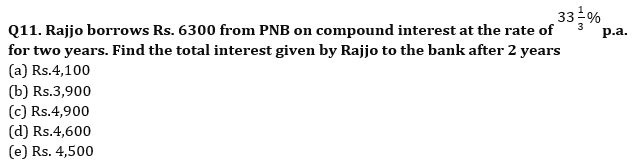Q12.The interest earned on an amount after 2 yrs at 10 % per annum compounded yearly is Rs 672. Find the interest earned on same amount after 4 yr at 14 % per annum at simple interest ?
(a) Rs 1792
(b) Rs 1864
(c) Rs 1912
(d) Rs 1754
(e) Rs 1720

Q13. Difference between CI and SI on a sum for 3 year at 20% p.a. is 176, Find the simple interest on the sum after 2 year at 10% p.a.?
(a) 225
(b) 250
(c) 275
(d) 300
(e) 350

Q14. A man received Rs.3456 when he invested Rs. P at 12% p.a. at SI for 3 years. If he invested Rs.(P + 4400) at 15% p.a. at CI compounding annually for 2 years, then find the interest received by him.
(a) Rs.4515
(b) Rs.4960
(c) Rs.4725
(d) Rs.4185
(e) Rs.4345

Q15. Difference of the compound interest received in first year and second year at 20% per annum at CI is Rs 1200 then find the sum?
(a) Rs 25,000
(b) Rs 36,000
(c) Rs 35,000
(d) Rs 24,000
(e) Rs 30,000

Solutions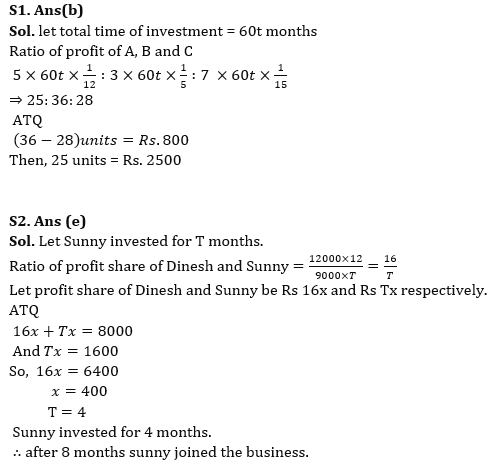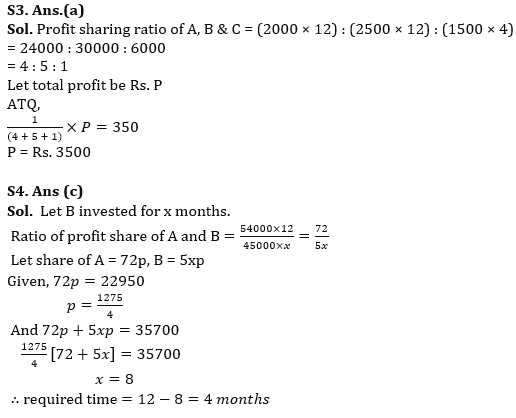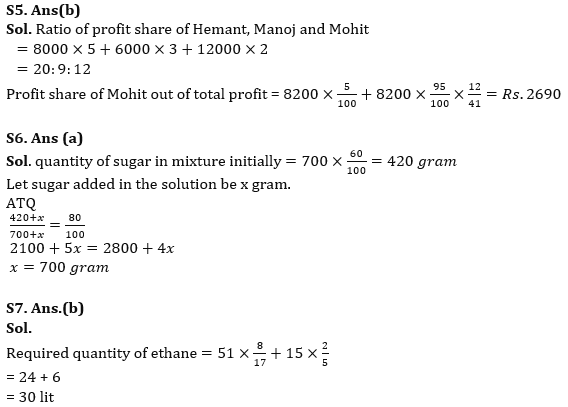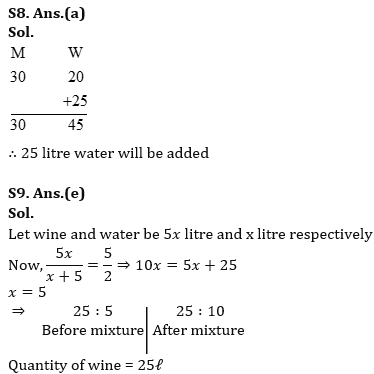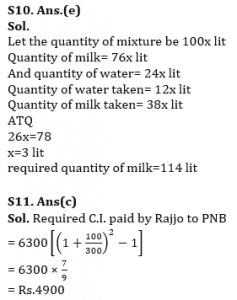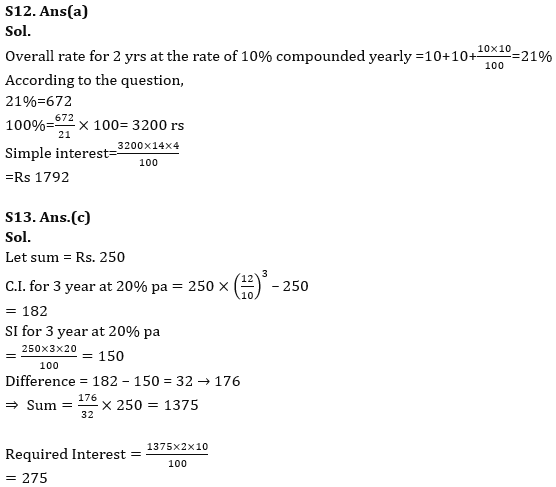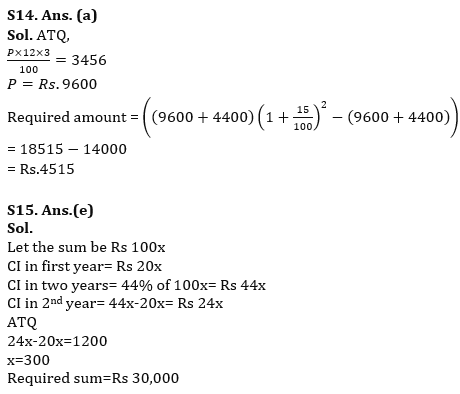## FAQs

### How many sections are there in the IRDAI exam?

There are 4 sections in the IRDAI assistant manager online preliminary exam. They are Reasoning, English Language, General awareness, Quantitative aptitude.

#### Congratulations!Union Budget 2023-24: Free PDF